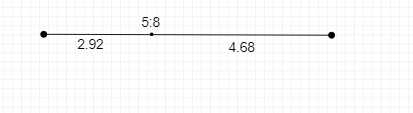# Draw a line segment of length 7.6 cm and divide it in the ratio 5:8. Measure the two parts.

Given :

The line segment of 7.6 cm  is divided in the ratio of 5:8.

To do :

We have draw the line segment of length 7.6 cm, and divide it in the ratio of 5:8

Solution :The line segment of 7.6 cm  is divided in the ratio of 5:8

Therefore, the two parts are,

$\frac{5}{8+5} \times 7.6\ cm$

$=\frac{5}{13} \times 7.6\ cm$

$=\ 2.92\ cm$

And

$\frac{8}{8+5} \times 7.6\ cm$

$=\frac{8}{13} \times 7.6\ cm$

$=4.68\ cm$